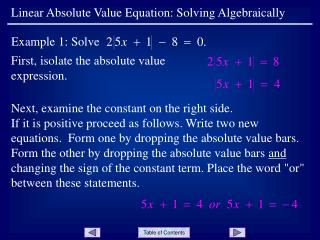DownloadDownload PresentationFirst, isolate the absolute value expression.

# First, isolate the absolute value expression.

Télécharger la présentation## First, isolate the absolute value expression.

- - - - - - - - - - - - - - - - - - - - - - - - - - - E N D - - - - - - - - - - - - - - - - - - - - - - - - - - -
##### Presentation Transcript

1. Example 1: Solve Linear Absolute Value Equation: Solving Algebraically First, isolate the absolute value expression. Next, examine the constant on the right side. If it is positive proceed as follows. Write two new equations. Form one by dropping the absolute value bars. Form the other by dropping the absolute value bars and changing the sign of the constant term. Place the word "or" between these statements.

2. The solution set is Linear Absolute Value Equation: Solving Algebraically Now solve each equation. The "or" means that the solution of each equation is a solution of the original equation. See next slide for notes. Slide 2

3. Linear Absolute Value Equation: Solving Algebraically Notes: If after isolating the absolute value expression, the constant term on the right is negative, the equation would have no solution because any real number substituted for x would cause the absolute value expression to produce a nonnegative number. If after isolating the absolute value expression, the constant term on the right is zero, remove the absolute value bars and solve the resulting equation. For example, solving |2x + 3| = 0 would result in solving 2x + 3 = 0. Slide 3

4. Linear Absolute Value Equation: Solving Algebraically END OF PRESENTATION Click to rerun the slideshow.